## 尊敬的用户：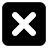### 三年级数学上册第四章《乘与除》小树有多少棵—整十整百的数乘一位数的口算

• 同步课程
• 课后作业
• 同步试卷
• 本节重点
• 主讲老师

2×3=6
=3+3
=2+2+2

3×9=9+9+9
20×4=20+20+20+20

1.口算：
3×8=  5×4=  9×3=
4×2=  8×7=  7×9=
5×5=  8×4=  6×7=
2.口答：
（1）3个十是多少？15个十呢？
15个百呢？
（2）80是几个十？600是几个百？900是几个百？

3捆小树一共有多少棵？
20×3=()(棵)

4捆小树一共有多少棵？
20×4=80（棵）
5捆小树一共有多少棵？
20×5=100（棵）
3×2=6   2×4=8   2×5=10
3×20=60 20×4=80 2×50=100

500×3=
500+500+500=1500
5×3=15
500×3=1500

3×200=600   2×400=800   200×5=1000
4×300=1200  400×4=1600  3×500=1500

8×4=32
80×4=320
800×4=3200
6×7=42
6×70=420
6×700=4200

4×6=24
4×60=240
4×600=2400

1、30×4  50×8  9×600
40×5  60×7  800×4
2、70×8  30×6  600×9
4×60  20×7  3×800
90×5  8×50  700×4

（1）3×80=240（√）
（2）50×6=30（×）
（3）2×500=100（×）

1.每种水果分别有多少个？算一算，说说你是怎样想的。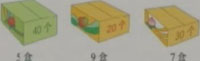5×40=200（个）  9×20=180（个） 7×30=210（个）
2.算一算，说一说你是怎样想的。
70×8=560  30×6=180  600×9=5400
4×60=240  40×5=200  5×800=4000
3.4.看图列式计算。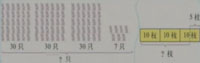3×30+7=97（只）
5.象爱吃香蕉。

（1）一堆香蕉够3头小象吃1天吗？
（2）1头大象一个星期吃多少根香蕉？
（3）一堆香蕉供3头象吃1天，结果还差10根。你知道其中有几头大象和几头小象吗？
（1）60×3=180（根）
180＜200

（2）90×7=630（根）
（3）200+10=210（根）
60×2+90=210（根）

## 一、填空题

1．填一填。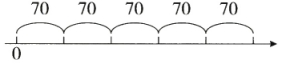×=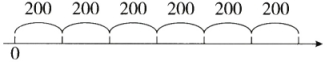×=

【参考答案】：70、5、350、200、6、1200

## 二、判断题

1．判断。
(1)算式30×4和3×40的计算结果相同，表示的意义也相同。（

(2)计算20个5相加的和，可以写成20×5，也可以写成5×20。（

(3)因为800的末尾有两个0，所以计算800×5时，乘积的末尾也应该只有两个0。（

## 三、主观题

1．开学了，王老师要为三年级学生重新注册学生卡。可是三(2)班和三(4)班各有一名学生丢失了学生卡，请你帮忙算一算。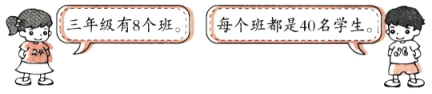(1)王老师应该收上来多少张学生卡？
(2)王老师实际能收上来多少张学生卡？

【参考答案】：(1)40×8=320（张）

(2)320-(1+1)=318（张）

2．李明一家人邀请王越一家人一同到野生动物园游玩。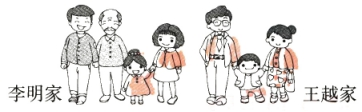(1)购买成人票需要花多少元？
(2)李明的爸爸准备了600元，买门票够吗？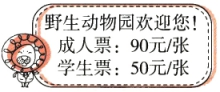【参考答案】：(1)90×5=450（元）

(2)50×2+450=550（元）  550元＜600元

3．李老师在文体店买了足球和篮球共8个，一共花了470元，李老师买了几个足球和几个篮球？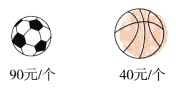【试题解析】：这是一道综合题，需要运用整十数乘一位数以及三位数加法的知识，同时需要有序思考问题。可以依次按照7个篮球+1个足球（370元）、6个篮球+2个足球（420元）、5个篮球+3个足球（470元）尝试计算。也可以运用估算的知识，5个足球已经45O元，很接近470元了，再买1个篮球就已经450+40=490（元），与题目不符；如果买4个足球花90×4=360（元），还剩470-360=110（元）不能正好买整数个篮球，也不对；如果买3个足球花90×3=270（元），还剩470-270=200（元），而恰好40×5=200(元)，即买3个足球和5个篮球是正确的。也可以通过列表格的方法，清晰地表示思考过程。
 篮球钱数 足球钱数 总钱数 40×7=280(元) 90×1=90(元) 280+90=370(元) 40×6=240(元) 90×2=180(元) 240+180=420(元) 40×5=200(元) 90×3=270(元) 200+270=470(元)

【参考答案】：40×5=200（元）  90×3=270（元）
200+270=470（元）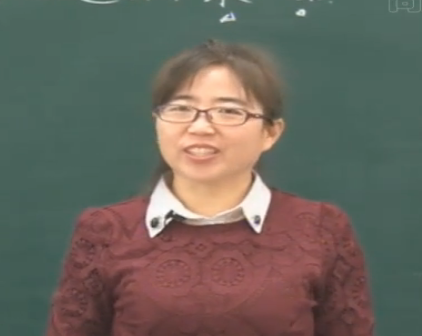©2016 同桌100 All Rights Reserved